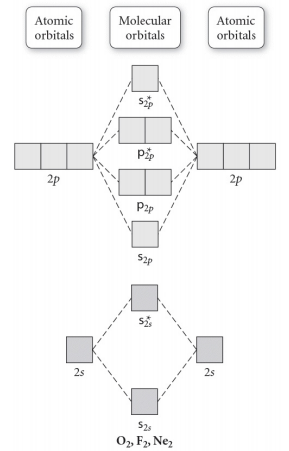# Problem: Use the molecular orbital diagram shown to determine which of the following is MOST stable. In molecular orbital names, s = sigma and p = pi.a. F2b. F22-c. O22+d. F22+e. Ne22+

###### FREE Expert Solution

Construct the molecular orbital diagram for each molecule and determine which is most stable by counting the electrons in anti-bonding MOs.

Recall that as the electrons in the anti-bonding MO (denoted by *) increases, the more unstable the compound is.

For this matter, we have to look for the molecule with the least amount of electrons in the antibonding MO

We need to look at the molecular orbital (MO) diagram for each molecule; to do so:

Step 1: Calculate the total number of valence electrons present.

Step 2: Draw the molecular orbital diagram.

Recall that the bonding MOs are those without an asterisk (e.g., σ1s), while the antibonding MOs are those with an asterisk (e.g., σ1s*).

100% (469 ratings)###### Problem Details

Use the molecular orbital diagram shown to determine which of the following is MOST stable. In molecular orbital names, s = sigma and p = pi.a. F2

b. F22-

c. O22+

d. F22+

e. Ne22+